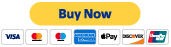Search

# Twin Primes - A more formal proof.

Updated: Dec 26, 2019

Here is a more formal statement of the proof with formulae given in LaTex format. The wording could be improved a bit more, or a few formulas added between lines for clarity, however this should be enough for mathematicians familiar with the topic to discuss the concept.

**(Update)**

Given there are infinite primes.

Given $z\neq xy$ is the set of primes for $1<x$ and $1<y$.

Therefore, there are infinite holes, values with no integer solutions, on that surface.

Given there are an infinite number of primes 2 away from some number.

Given $z\neq xy-2$ checks the primality of a number $z+2$ for $1<x$ and $1<y$. This is the first surface slid down by 2.

Therefore, there are infinite holes on the second surface.

The Conjecture is now stated as to ask if there are infinite elements in the intersection of those 2 infinite sets.

Given if there is an integer x, such that $x=\frac{b\pm \sqrt{b^{2}-4z}}{2}$ for $2\sqrt{z}\leq b\leq \left \lfloor \left ( \frac{z}{2}+2 \right ) \right \rfloor$, then z is composite.

For the second surface, $2\sqrt{z+2}\leq b\leq \left \lfloor \left ( \frac{z+2}{2}+2 \right ) \right \rfloor$.

Therefore, there are an infinite number of solutions where x is not an integer.

Therefore, $b\pm \sqrt{b^{2}-4z}\neq even$.

Therefore, when b is even the square root must be odd, and when b is odd the square root must be even.

Therefore, the square root must not equal an integer.

Therefore, $4z$ and $4z-8$ must not equal a difference of squares.

Therefore, there are infinite spots where $4z$ and $4z-8$ do not equal a difference of squares respectively.

Given a difference of squares $x^{2}-\left ( x-y \right )^{2}$.

Therefore, $y\neq x\pm \sqrt{x^{2}-4z}$.

Therefore, $\sqrt{x^{2}-4z}\neq -x+b$ for $\sqrt{4z}\leq b\leq \infty$.

Therefore, $z\neq \frac{-b^{2}+2xb}{4}$, which I like to rewrite for convention as $z\neq \frac{-x^{2}+2yx}{4}$, has infinite solutions.

For surface 2, $z\neq \frac{-x^{2}+2yx+8}{4}$.

Therefore these surfaces represent the conditions to be checked.

Therefore, all values on these surfaces do not meet the conditions.

Therefore there are infinite values not on these surfaces that meet each's condition respectively.

Given, not all values not on these surfaces meet the conditions.

The Conjecture is now stated as to ask if infinite values, not on the surfaces, within the restrictions of the parameters, and that do meet both conditions, can be found.

Therefore for any odd x, $\frac {x^{2}+3}{4}$ will create a value that is not on either surface for the appropriate range of parameters.

Because there are infinite odd numbers, the parabola $\frac {x^{2}+3}{4}$ generates infinite such values.

Given the values are all odd composites, prime, or the second prime of a twin pair.

Given an odd composite $\left ( 2n+1 \right )\left ( 2m+1 \right )= 4nm+2n+2m+1$, then $\frac{x^{2}+3}{4} \neq \left ( 2n+1 \right )\left ( 2m+1 \right )= 4nm+2n+2m+1$.

Therefore, $x\neq \sqrt{16nm+8n+8m+1}$

Given that 2 less than a prime that is not a second twin is composite yields: $\frac{x^{2}+3}{4}-2 \neq \left ( 2n+1 \right )\left ( 2m+1 \right )= 4nm+2n+2m+1$.

Therefore, $x\neq \sqrt{16nm+8n+8m+9}$.

Therefore, as long as $x\sqrt{16nm+8n+8m+1}$ and $x\sqrt{16nm+8n+8m+9}$ do not sweep out the set of all odds, which they do not, then there are infinite values that meet both conditions.

Therefore, there are infinite Twin Primes. **(end edit)**

16 views

### Recent Posts

See All

#### Simplified Proof of the Twin Primes Conjecture© 2017- 2020 by Thoughtfarm. Proudly created with Wix.com